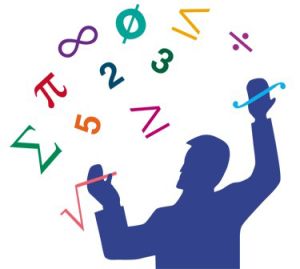# Mathematics Knowledge Trivia Quiz

15 QuestionsSettingsThe quiz below consists of different math problems and it is designed to test your mathematics knowledge. It is for the brave and if you are too scared do not attempt it. Give it a try and share your score in the comment section. Hope you get the highest and good luck!

Related Topics
• 1.
If Tony pays \$250 per month for his apartment rent, which equation shows how much he will pay in the next 12 months?
• A.

\$250 - \$20

• B.

\$250 + \$12

• C.

\$250 x \$12

• D.

\$250 x \$250

• 2.
(4 + 1.3) x 2.1 =
• A.

-11.13

• B.

11.13

• C.

12.14

• D.

22.21

• 3.
7.5 + 6.8 =
• A.

10.2

• B.

14.3

• C.

18.4

• D.

21.9

• 4.
0.1 x -1.8 =
• A.

-0.18

• B.

-0.08

• C.

1.18

• D.

-1.18

• 5.
7.7 - 4.1 =
• A.

2.88

• B.

3.6

• C.

5.5

• D.

11.3

• 6.
6.28 + 1.8 =
• A.

8.08

• B.

10.21

• C.

11.10

• D.

12.09

• 7.
A trapezoid has a top base of 4 inches, bottom base of 6 inches, and a height of 5 inches.  What is the area of the trapezoid in square inches?
• A.

20

• B.

25

• C.

31

• D.

44

• 8.
-3.5 x -1.2 =
• A.

4.2

• B.

6.3

• C.

8.85

• D.

12.11

• 9.
Which number sentence is true?
• A.

62

• B.

0.62 < 0.062

• C.

62.62 < 6.262

• D.

.006262 < 0.062

• 10.
Which of the following means 3n + 7 = 16
• A.

7 more than 3 times a number is 16

• B.

3 more than 7 times a number is 16

• C.

7 less than 3 times a number is 16

• D.

10 times a number is 16

• 11.
Which of the following is the equivalent of 12 divided by 25?
• A.

.22

• B.

.48

• C.

.65

• D.

1.12

• 12.
25 percent of what number is equal to 5?
• A.

2

• B.

10

• C.

20

• D.

50

• 13.
Convert 0.25 to a fraction:
• A.

1/2

• B.

1/4

• C.

1/8

• D.

1/10

• 14.
The three angles of a triangle are 3x, x + 10, and 2x - 40.  Find the size of the smallest angle in the triangle:
• A.

25

• B.

30

• C.

45

• D.

55

• 15.
Factor completely:  2 x 2 - 2x - 12
• A.

(2x+3) (x-2)

• B.

(2x+4)(x-3)

• C.

(2x+3)(x-4)

• D.

(2x-7)(x-5)